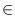Next: Rational Circles ( rat_circle Up: Basic Data Types for Previous: Rational Rays ( rat_ray   Contents   Index

# Straight Rational Lines ( rat_line )

Definition

An instance l of the data type ratline is a directed straight line in the two-dimensional plane.

#include < LEDA/geo/rat_line.h >

Types

 rat_line::coord_type the coordinate type (rational). rat_line::point_type the point type (rat_point). rat_line::float_type the corresponding floatin-point type (line).

Creation

 rat_line l(const rat_point& p, const rat_point& q) introduces a variable l of type rat_line. l is initialized to the line passing through points p and q directed form p to q. Precondition pq. rat_line l(const rat_segment& s) introduces a variable l of type rat_line. l is initialized to the line supporting segment s. Precondition s is nontrivial. rat_line l(const rat_point& p, const rat_vector& v) introduces a variable l of type rat_line. l is initialized to the line passing through points p and p + v. Precondition v is a nonzero vector. rat_line l(const rat_ray& r) introduces a variable l of type rat_line. l is initialized to the line supporting ray r. rat_line l introduces a variable l of type rat_line. rat_line l(const line& l1, int prec = rat_point::default_precision) introduces a variable l of type rat_line. l is initialized to the line obtained by approximating the two defining points of l1.

Operations

 line l.to_float() returns a floating point approximation of l. void l.normalize() simplifies the homogenous representation by calling point1().normalize() and point2().normlize(). rat_point l.point1() returns a point on l. rat_point l.point2() returns a second point on l. rat_segment l.seg() returns a segment on l. bool l.is_vertical() decides whether l is vertical. bool l.is_horizontal() decides whether l is horizontal. rational l.slope() returns the slope of s. Precondition l is not vertical. rational l.x_proj(rational y) returns p.xcoord(), where pline(l) with p.ycoord() = y. Precondition l is not horizontal. rational l.y_proj(rational x) returns p.ycoord(), where pline(l) with p.xcoord() = x. Precondition l is not vertical. rational l.y_abs() returns the y-abscissa of line(l ), i.e., l.y_proj(0). Precondition l is not vertical. bool l.intersection(const rat_line& g, rat_point& inter) returns true if l and g intersect. In case of intersection a common point is returned in inter. bool l.intersection(const rat_segment& s, rat_point& inter) returns true if l and s intersect. In case of intersection a common point is returned in inter. bool l.intersection(const rat_segment& s) returns true, if l and s intersect, false otherwise. rat_line l.translate(const rational& dx, const rational& dy) returns l translated by vector (dx, dy). rat_line l.translate(integer dx, integer dy, integer dw) returns l translated by vector (dx/dw, dy/dw). rat_line l.translate(const rat_vector& v) returns l translated by vector v. Precondition v.dim() = 2. rat_line l + const rat_vector& v returns l translated by vector v. rat_line l - const rat_vector& v returns l translated by vector - v. rat_line l.rotate90(const rat_point& q, int i=1) returns l rotated about q by an angle of i x 90 degrees. If i > 0 the rotation is counter-clockwise otherwise it is clockwise. rat_line l.reflect(const rat_point& p, const rat_point& q) returns l reflected across the straight line passing through p and q. rat_line l.reflect(const rat_point& p) returns l reflected across point p. rat_line l.reverse() returns l reversed. rational l.sqr_dist(const rat_point& q) returns the square of the distance between l and q. rat_segment l.perpendicular(const rat_point& p) returns the segment perpendicular to l with source p and target on l. rat_point l.dual() returns the point dual to l. Precondition l is not vertical. int l.orientation(const rat_point& p) computes orientation(a, b, p), where ab and a and b appear in this order on line l. int l.side_of(const rat_point& p) computes orientation(a, b, p), where ab and a and b appear in this order on line l. bool l.contains(const rat_point& p) returns true if p lies on l. bool l.clip(rat_point p, rat_point q, rat_segment& s) clips l at the rectangle R defined by p and q. Returns true if the intersection of R and l is non-empty and returns false otherwise. If the intersection is non-empty the intersection is assigned to s; It is guaranteed that the source node of s is no larger than its target node. bool l == const rat_line& g returns true if the l and g are equal as oriented lines. bool equal_as_sets(const rat_line& l, const rat_line& g) returns true if the l and g are equal as unoriented lines.

Non-Member Functions

 int orientation(const rat_line& l, const rat_point& p) computes orientation(a, b, p), where ab and a and b appear in this order on line l. int cmp_slopes(const rat_line& l1, const rat_line& l2) returns compare(slope(l1), slope(l2)). rat_line p_bisector(const rat_point& p, const rat_point& q) returns the perpendicular bisector of p and q. The bisector has p on its left. Precondition pq.Next: Rational Circles ( rat_circle Up: Basic Data Types for Previous: Rational Rays ( rat_ray   Contents   Index